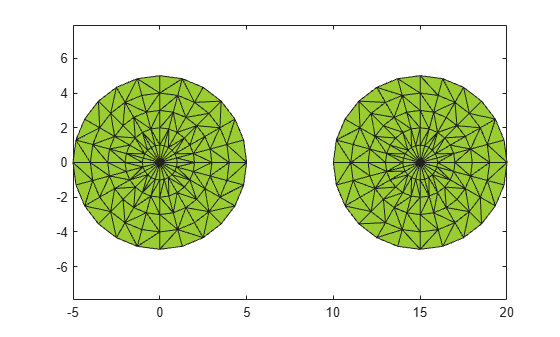# area

Area of 2-D alpha shape

## Syntax

``A = area(shp)``
``A = area(shp,RegionID)``

## Description

example

````A = area(shp)` returns the area of 2-D alpha shape `shp`.```

example

````A = area(shp,RegionID)` returns the area of a region of the alpha shape. `RegionID` is the ID for the region and `1` ≤ `RegionID` ≤ `numRegions(shp)`.```

## Examples

collapse all

Create a set of 2-D points.

```th = (pi/12:pi/12:2*pi)'; x1 = [reshape(cos(th)*(1:5), numel(cos(th)*(1:5)),1); 0]; y1 = [reshape(sin(th)*(1:5), numel(sin(th)*(1:5)),1); 0]; x = [x1; x1+15;]; y = [y1; y1];```

Create and plot an alpha shape using an alpha radius of 2.5.

```shp = alphaShape(x,y,2.5); plot(shp)```Compute the area of the alpha shape.

`totalarea = area(shp)`
```totalarea = 155.2914 ```

Compute the areas of each of the two regions separately.

`regionareas = area(shp, 1:numRegions(shp))`
```regionareas = 1×2 77.6457 77.6457 ```

## Input Arguments

collapse all

Alpha shape, specified as an `alphaShape` object. For more information, see `alphaShape`.

Example: `shp = alphaShape(x,y)` creates a 2-D `alphaShape` object from the `(x,y)` point coordinates.

ID number for region in alpha shape, specified as a positive integer scalar between `1` and `numRegions(shp)`.

An alpha shape can contain several smaller regions, depending on the point set and parameters. Each of these smaller regions is assigned a unique `RegionID`, which numbers the regions from the largest area or volume to the smallest. For example, consider a 3-D alpha shape with two regions. The region with the largest volume has a `RegionID` of 1, and the smaller region has a `RegionID` of 2.

Example: `shp.RegionThreshold = area(shp,numRegions(shp)-2);` suppresses the two smallest regions in 2-D alpha shape `shp`.

Data Types: `double`

## Version History

Introduced in R2014b